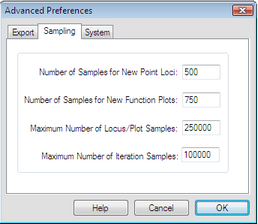﻿ Windows > Advanced Preferences > Sampling Preferences

# Sampling PreferencesHold the Shift key, choose and click the Sampling tab to open this panel.

Holding the Shift key changes the Preferences command to

The settings on this panel control the number of samples used for loci, function plots, and iterations. In general, the more samples, the more accurate or detailed the object appears, but the slower it is for Sketchpad to compute and draw. If your computer is faster than most, you may want to increase the values of these settings; if it’s slow to draw and recalculate loci, plots, and iterations you may want to decrease these values.

You can adjust the number of samples used in any specific locus or function plot by visiting its Plot Properties.

Number of samples for new point loci: This value determines the number of samples used in a newly created point locus. (The number of samples in a new locus of a nonpoint object is proportionate to, but smaller than, this number.)

Number of samples for new function plots: This value determines the number of samples used in a newly created function plot.

Maximum number of locus/plot samples: After you’ve created a locus or function plot, use Properties to change the number of samples. This value determines the largest number you can use in the Plot panel of the Properties dialog box when you’re changing the number of samples for such an object.

Maximum number of iteration samples: This value limits the number of samples allowed in an iterated image. For an iteration using a single map, this is the maximum number of iterations. For an iteration using more than one map, this value limits the depth in such a way that the total number of iterated images of a single object never exceeds this number.